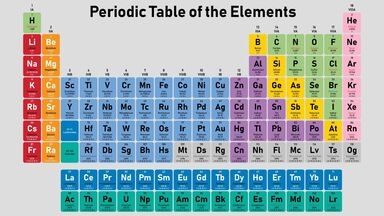# Examples of Ionization Energy

The term ionization energy is a reference to the quantity, or amount, of energy necessary to expel an electron from the gaseous form of an atom or molecule. As electrons are removed, it becomes more difficult to remove another because the charge of the atom has changed, and the electron is more attracted to stay with the atom. As such, different ionization energy can be required under different circumstances. Learn how to properly calculate the quantity of energy required and see ionization energy examples.Periodic Table as examples of ionization energy

## Sodium Ionization Energy Example

To see an ionization energy example at play, let's look at sodium. For this ionization energy example, Na stands for sodium and e- is the electron that is removed from the sodium atom.

First Ionization level (IE1):

Na(g) → Na+(g) + e-
IE1 = 496 kJ/mol

Second Ionization level (IE2):

Na+(g) → Na2+(g) + e
IE2 = 4560 kJ/mol

Third Ionization level (IE3):

Na2+(g) → Na3+(g) + e
IE3 = 6913 kJ/mol

### Ionization Energy Examples: Highest Levels

This table shows examples of the elements that require the most ionization energy at the first level of ionization.

 Atomic Number Name 1st Ionization Energy Level in Electron Volts (eV) or kilojoules/moles (kJ/mol) 2 helium 24.59 or 2372.3 10 neon 21.56 or 2080.7 9 fluorine 17.42 or 1681.0 18 argon 15.76 or 1520.6 7 nitrogen 14.53 or 1402.3 36 krypton 14 or 1350.8 8 oxygen 13.62 or 1313.9 17 chlorine 12.97 or 1250.3 54 xenon 12.13 or 1170.4 6 carbon 11.26 or 1086.5

To convert from eV to kJ/mol, multiply by 96.4689. Some numbers vary based on rounding.

### Ionization Energy Examples: Lowest Levels

Now that you've looked at the highest levels of ionization energy, explore the lowest ionization energy level examples.

 Atomic Number Name 1st Ionization Energy Level in Electron Volts (eV) or kilojoules/moles (kJ/mol) 55 cesium 3.89 or 375.7 87 francium 4.07 or 380 37 rubidium 4.18 or 403.0 19 potassium 4.34 or 418.8 103 lawrencium 4.9 or 470 89 actinium 5.17 or 499 56 barium 5.21 or 502.9 88 radium 5.28 or 509.3 3 lithium 5.39 or 520.2 71 lutetium 5.43 or 523.5

## Understanding Ionization Energy

Ionization energy increases moving from left to right on the periodic table, a table of the elements. The energy decreases moving from top to bottom on the periodic table.

Ionization of energy must be calculated for each ion on the periodic table. As such, to understand the ionization energy, it is helpful to understand the equations that are used in calculating the quantity of energy necessary to expel electrons.

### Basic Equation of Ionization Energy

The basic equation for ionization energy is:

X(g) → X+(g) + e-

The amount of energy necessary changes each time an electron is let go since it becomes more difficult to remove electrons after one or more has already been removed from the atom or molecule. Therefore, the equation changes.

The different equations for each ionization level are as follows:

• 1st ionization energy equation:

X(g) → X+(g) + e-

• 2nd ionization energy equation:

X+(g) → X2+(g) + e-

• 3rd ionization energy equation:

X2+(g) → X3+(g) + e-

### Ionization Units

Units used to measure ionization are not always the same.

• Chemists refer to one mole (mol) of a substance when reporting ionization energy. This unit is kJ/mol or kcal/mol.
• The unit electron volt (eV) is used by physicists.

## Ionization Energy At Work

It is important for those in various scientific fields, including chemistry and physics, to understand the concept of ionization energy and to understand how to use the equations to calculate the ionization energy for each element. The different equations for different ionization levels, as well as the information on the different ionizations of energy for each element, are helpful for those who are hoping to better understand how ionization energy works. To learn more about ionization, check out examples of ionization and the bonds it creates.